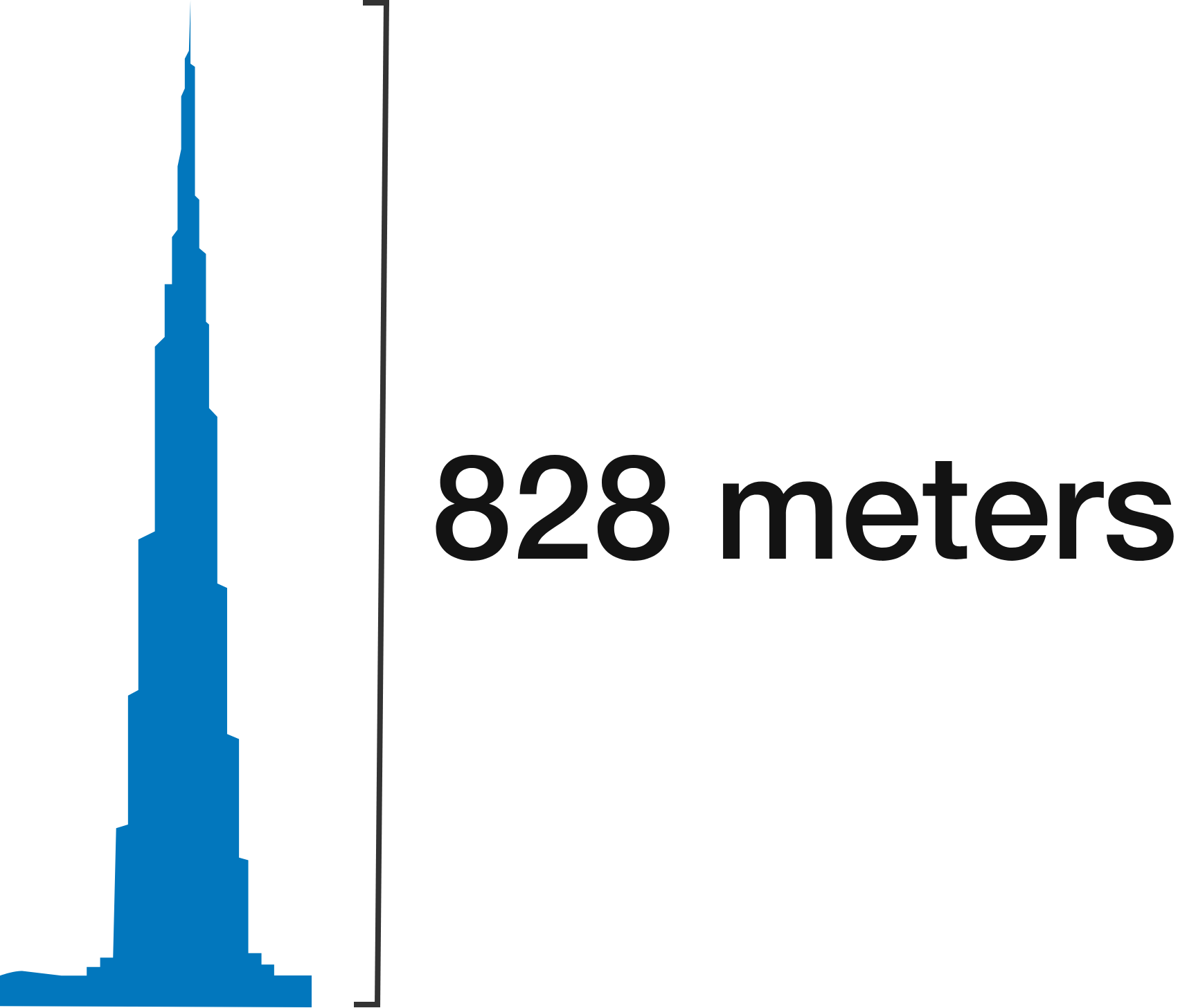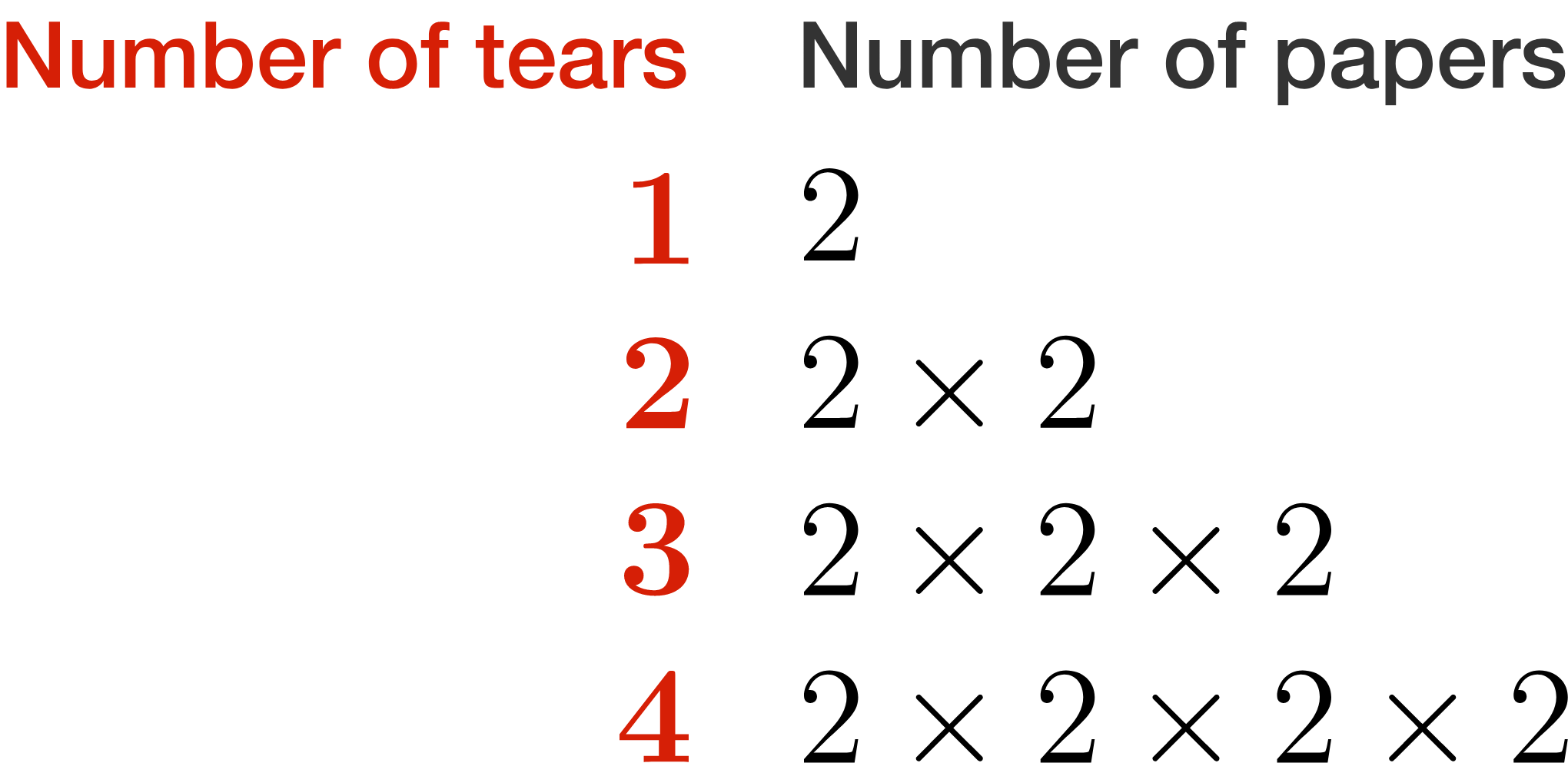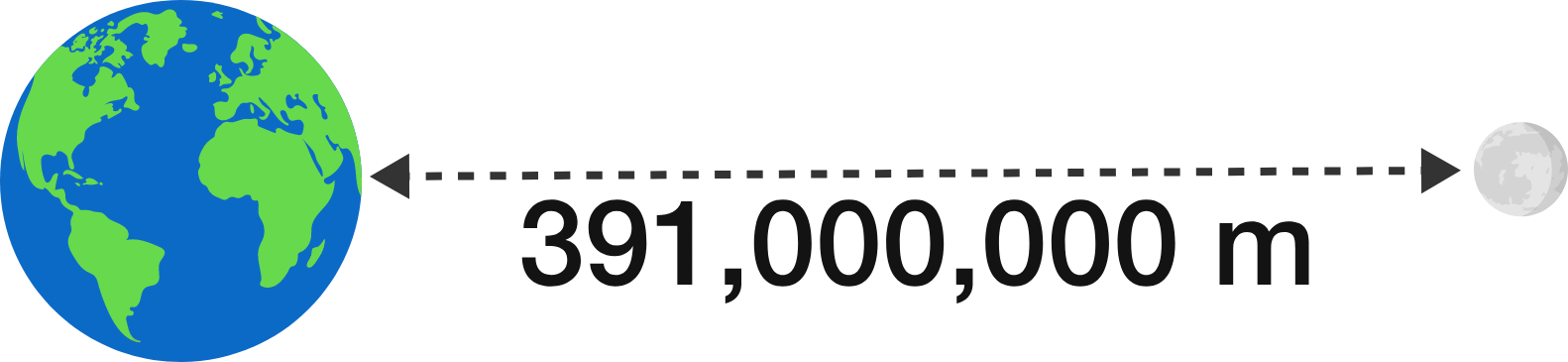### Pre-Calculus

Suppose you begin with a single sheet of paper.

You tear the paper in half and stack the pieces on top of each other. You now have two pieces of paper in a stack.

Repeat the process again with the stack you just obtained: tear the papers in half and stack them, and now after the second tear you have four pieces of paper.

If this can be repeated as many times as necessary (not worrying about paper size or how you get the papers to balance), and a stack of 100 papers is 1 cm, how many tears will it take until the height of the stack exceeds that of the Burj Khalifa, the tallest building in the world (at 828 meters)?

Just use your intuition: we'll get more exact in a moment!# Exponential Growth to the MoonThe pattern of tears multiplies the previous number of papers by 2, leading to the pattern above. $5$ tears, for instance, leads to $2 \times 2 \times 2 \times 2 \times 2 ,$ or more compactly, $2^5 .$

We can condense $2 \times 2 \times 2 \times \ldots \times 2$ being multiplied $n$ times into $2^n ,$ so $f(n) = 2^n$ is a function where $n$ is the number of tears and $f(n)$ is the number of papers. $f$ in this case is an exponential function.

To solve the previous problem exactly, then, we just need to know how many papers are needed to exceed the goal height of 828 meters, then figure out the smallest $n$ where $f(n)$ is large enough.

# Exponential Growth to the Moon

Time to get more exact: using the same tearing process as before, with 100 papers being 1 cm and a goal of exceeding 828 meters, how many tears are needed?

# Exponential Growth to the Moon

How many tears will it take to reach the moon, about 391 million meters away? (Again, use 100 papers as being 1 centimeter.)# Exponential Growth to the Moon

For the problems you just did, the method of solution involved sliding the value of $n$ until the answer reached our goal.

Exponential growth often causes this kind of surprising result, even when considering the vastly large orders of magnitude that come from space.

At the end, we solved the equation

$2^n = 3.91 \times 10^{12}$

but in a way that required we test out values using the sliders. We'd like a more general method of solving for the exponent which you'll get a taste of in the next quiz.

×